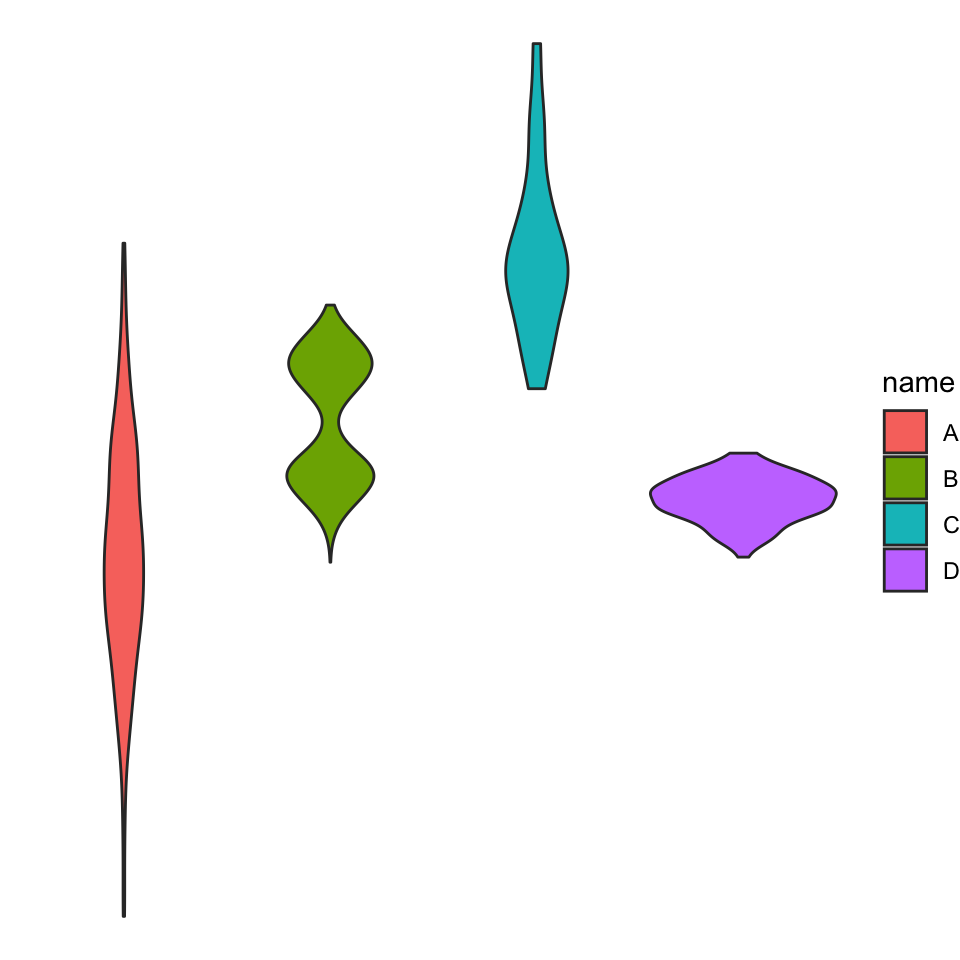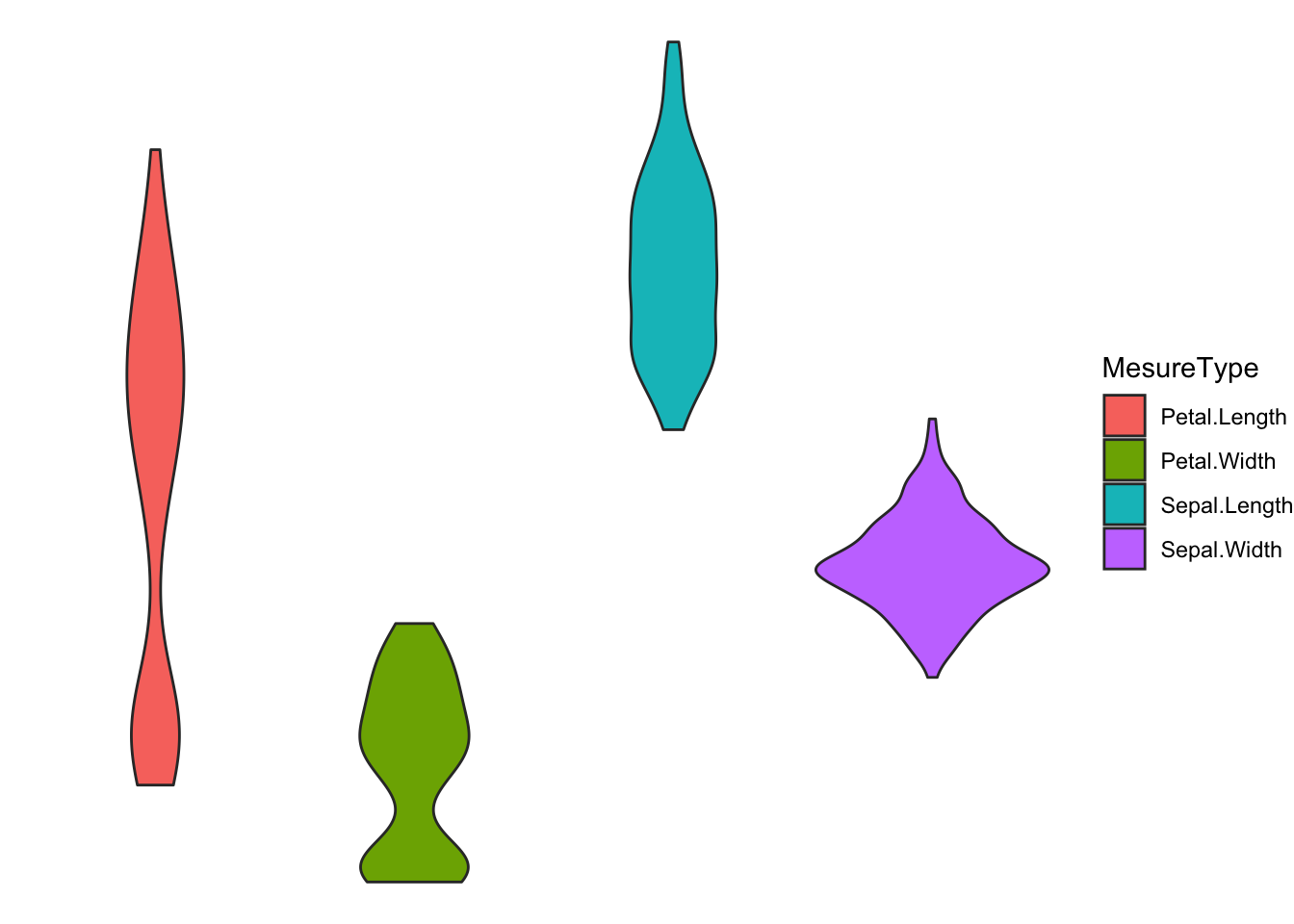# Most basic violin plot with ggplot2

A violin plot allows to compare the distribution of several groups by displaying their densities. See how to build it with `R` and `ggplot2` below. Learn more about violin chart theory in data-to-viz.

# Basic violin plot

Building a violin plot with `ggplot2` is pretty straightforward thanks to the dedicated `geom_violin()` function.

``````# Library
library(ggplot2)

# create a dataset
data <- data.frame(
name=c( rep("A",500), rep("B",500), rep("B",500), rep("C",20), rep('D', 100)  ),
value=c( rnorm(500, 10, 5), rnorm(500, 13, 1), rnorm(500, 18, 1), rnorm(20, 25, 4), rnorm(100, 12, 1) )
)

# Most basic violin chart
p <- ggplot(data, aes(x=name, y=value, fill=name)) + # fill=name allow to automatically dedicate a color for each group
geom_violin()

#p``````# Note on input format

Ggplot2 expects input data to be in a long format: each row is dedicated to one observation. Your input needs 2 column:
- a categorical variable for the X axis: it needs to be have the class `factor`
- a numeric variable for the Y axis: it needs to have the class `numeric`

### → From long format

You already have the good format. It’s going to be a breeze to plot it with `geom_violin()` as follow:

``````# Library
library(ggplot2)
library(dplyr)

# Create data
data <- data.frame(
name=c( rep("A",500), rep("B",500), rep("B",500), rep("C",20), rep('D', 100)  ),
value=c( rnorm(500, 10, 5), rnorm(500, 13, 1), rnorm(500, 18, 1), rnorm(20, 25, 4), rnorm(100, 12, 1) ) %>% round(2)
)``````
name value
A 11.02
A 5.54
A 18.05
A 6.57
``````# Basic violin
ggplot(data, aes(x=name, y=value, fill=name)) +
geom_violin()``````### → From wide format

In this case we need to reformat the input. This is possible thanks to the `gather()` function of the `tidyr` library that is part of the `tidyverse`.

``````# Let's use the iris dataset as an example:
data_wide <- iris[ , 1:4]``````
Sepal.Length Sepal.Width Petal.Length Petal.Width
5.1 3.5 1.4 0.2
4.9 3.0 1.4 0.2
4.7 3.2 1.3 0.2
4.6 3.1 1.5 0.2
``````library(tidyr)
library(ggplot2)
library(dplyr)
data_wide %>%
gather(key="MesureType", value="Val") %>%
ggplot( aes(x=MesureType, y=Val, fill=MesureType)) +
geom_violin()``````Related chart types

## Contact

This document is a work by Yan Holtz. Any feedback is highly encouraged. You can fill an issue on Github, drop me a message on Twitter, or send an email pasting yan.holtz.data with gmail.com.